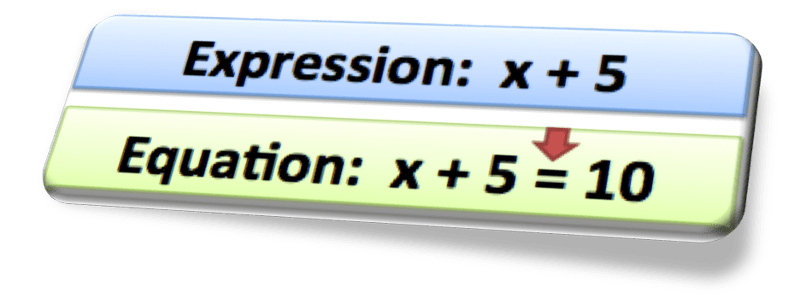# Equations and Their Solutions

In our everyday lives, we use equations to figure out different things such as:

How much money do I need to save to buy the game that is \$24 if I already have \$5 saved?

We use mental math to solve questions. However, not all problems can be solved using mental math. Some equations require more steps to solve for the missing piece of information. In this lesson, we are going to take a look at the beginning steps of equations.### What is an Equation?

An equation is a mathematical sentence that contains an equals sign. If an equation contains variables, a value for the variable that makes the equation true is a solution to the equation.   To test if a value is a solution of an equation, substitute the value for the variable.

An equation is a grouping of numbers, operations, and variables. This sounds very similar to an expression. However, the difference is that in an expression the variable can be any value, while in an equation a variable has a specific value and only one value.

## Practice

Determine whether the given value of the variable is a solution.
1. 93 = 48 + v for v = 35
2. 112 = 14 x e for e = 8
3. 13 = n ÷ 8 for n = 104
4. 79 = y – 23 for y = 112
5. 64 = m – 34 for m = 98
6. 105 = q x 7 for q = 14
7. 94 ÷ r = 26 for r = 3
8. w + 79 = 167 for w = 88
9. p + 36 = 54 for p = 18
10. x – 35 = 96 for x = 112
11. 12x = 84 for x = 7
12. 7y = 56 for y = 8

(source)